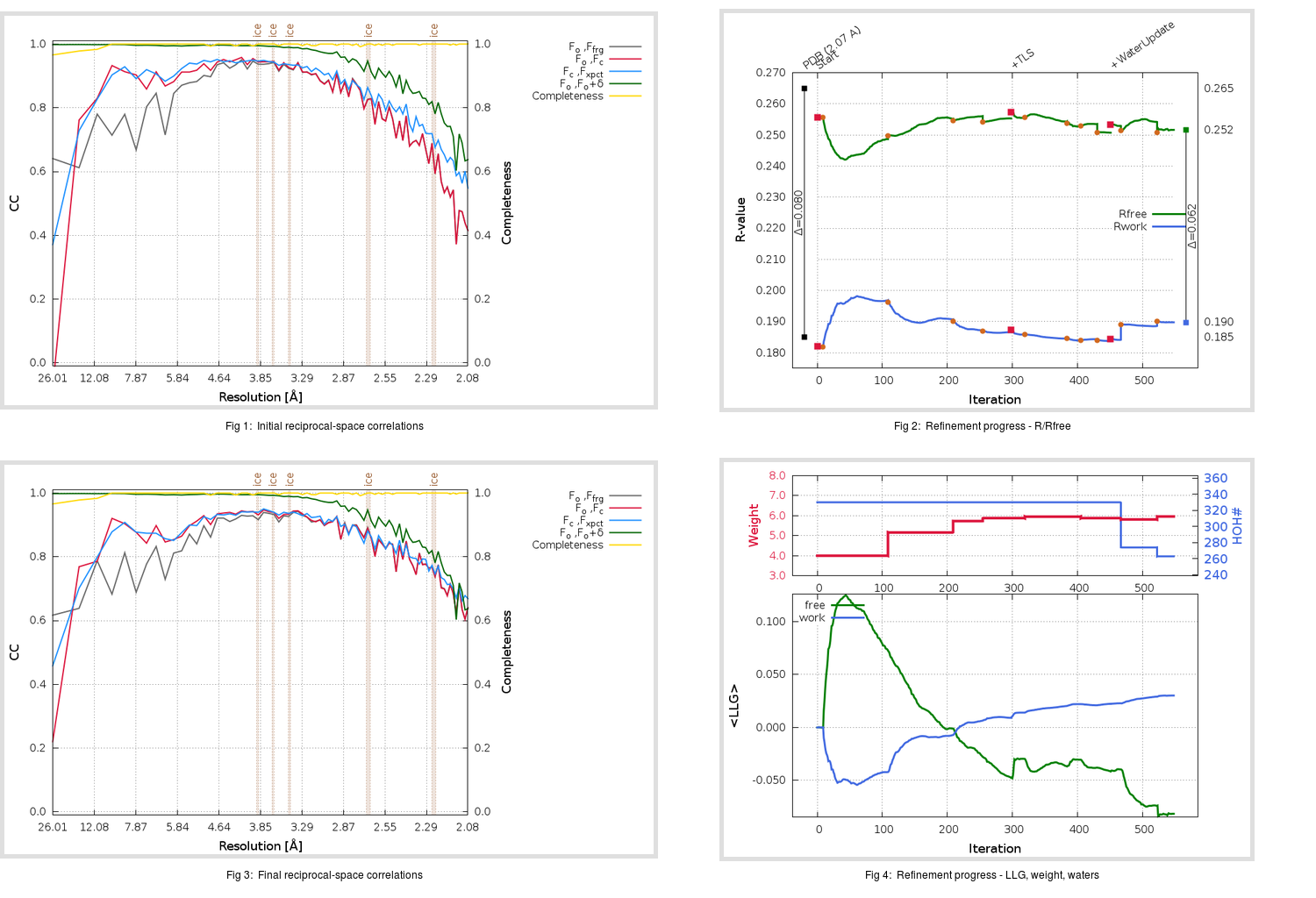Content:

## Deposited

` `
 Date deposited Date data collection Resolution R, Rfree 20200315 20200226 2.07 0.1810 0.2650

Molprobity (CCP4 7.0 version) summary:

```Ramachandran outliers =   0.66 %
favored =  95.03 %
Rotamer outliers      =   1.89 %
C-beta deviations     =     0
Clashscore            =   6.12
RMS(bonds)            =   0.0134
RMS(angles)           =   1.84
MolProbity score      =   1.89
Resolution            =   2.07
R-work                =   0.1810
R-free                =   0.2650
```

```Number of waters      =   330

<B> (all atoms) =   38.61 ( sd =   12.16 ) for       2730 non-hydrogen atoms
<B>   (protein) =   37.49 ( sd =   11.52 ) for       2370 non-hydrogen atoms
<B>     (water) =   45.96 ( sd =   13.34 ) for        330 non-hydrogen atoms
<B>    (others) =   47.53 ( sd =   20.14 ) for         30 non-hydrogen atoms

B min/max       (all non-hydrogen atoms) =   19.07 /  125.51
B min/max   (protein non-hydrogen atoms) =   20.70 /  113.23
B min/max     (water non-hydrogen atoms) =   19.07 /  125.51
B min/max     (other non-hydrogen atoms) =   34.95 /   73.85
```

## BUSTER (re-)refinement

` `

Molprobity (CCP4 7.0 version) summary:

```Ramachandran outliers =   0.33 %
favored =  97.35 %
Rotamer outliers      =   1.14 %
C-beta deviations     =     0
Clashscore            =   3.17
RMS(bonds)            =   0.0112
RMS(angles)           =   1.57
MolProbity score      =   1.28
Resolution            =   2.07
R-work                =   0.1898
R-free                =   0.2517
```

```Number of waters      =   263

<B> (all atoms) =   37.06 ( sd =    8.68 ) for       2663 non-hydrogen atoms
<B>   (protein) =   36.11 ( sd =    7.67 ) for       2370 non-hydrogen atoms
<B>     (water) =   44.84 ( sd =   11.54 ) for        263 non-hydrogen atoms
<B>    (others) =   44.74 ( sd =   19.05 ) for         30 non-hydrogen atoms

B min/max       (all non-hydrogen atoms) =   19.93 /  138.35
B min/max   (protein non-hydrogen atoms) =   24.42 /   74.38
B min/max     (water non-hydrogen atoms) =   19.93 /  138.35
B min/max     (other non-hydrogen atoms) =   34.29 /   64.89
```

Refinement progression:Results:

` `
 File Remark 5RE5_aB_refine.01_03_refine.pdb.gz exact refinement commands are in header 5RE5_aB_refine.01_03_refine.mtz.gz including original deposited data and several re-refinement map coefficients 5RE5_aB_refine.01_03_BUSTER_model.cif.gz including any non-standard compound restraints 5RE5_aB_refine.01_03_BUSTER_refln.cif.gz